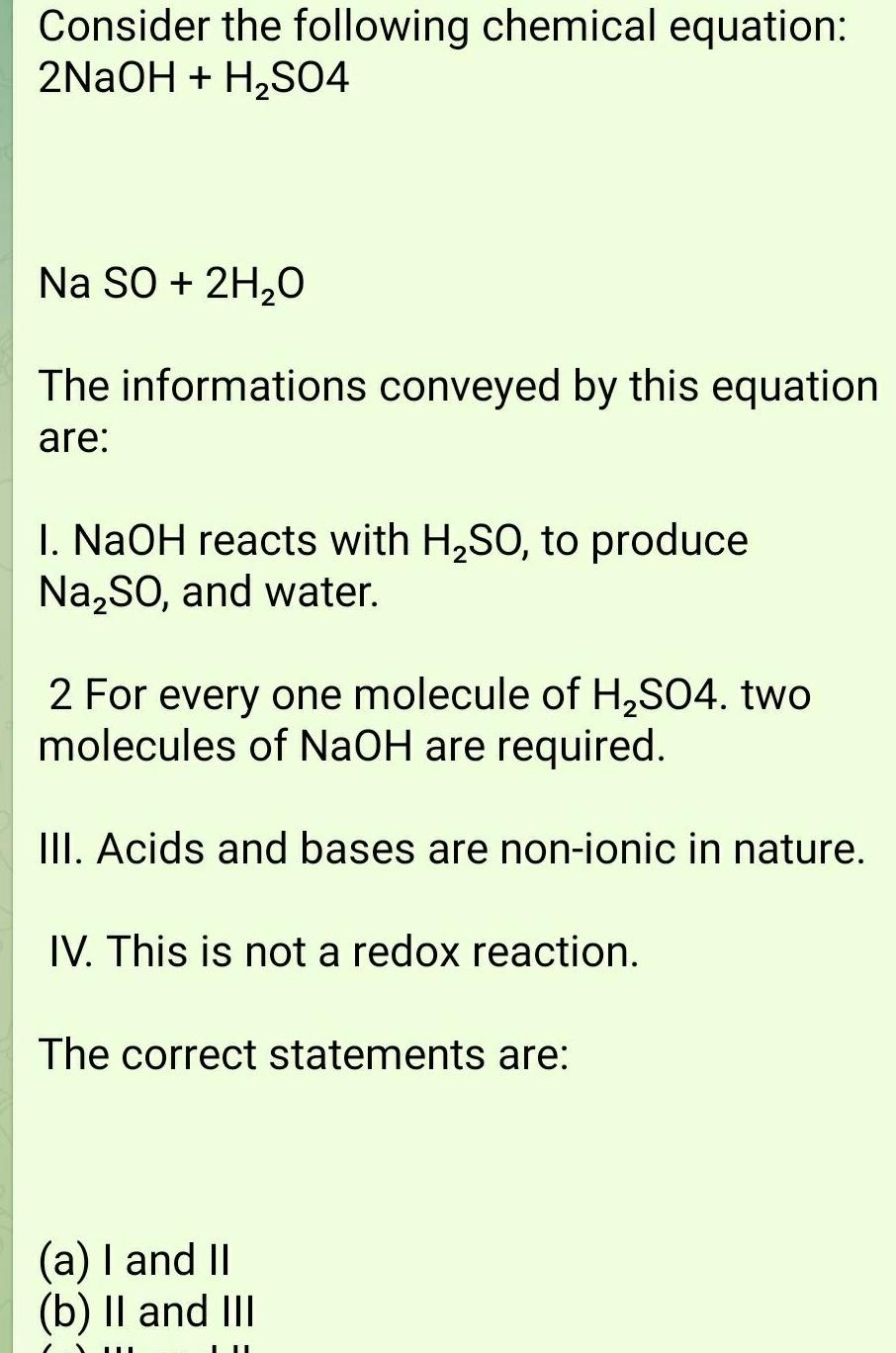Question:

# Consider the following chemical equation 2NaOH H SO4 Na SO

Last updated: 6/28/2023Consider the following chemical equation 2NaOH H SO4 Na SO 2H O The informations conveyed by this equation are 1 NaOH reacts with H SO to produce Na SO and water 2 For every one molecule of H SO4 two molecules of NaOH are required III Acids and bases are non ionic in nature IV This is not a redox reaction The correct statements are a I and II b II and III